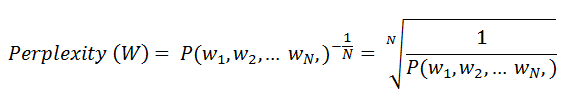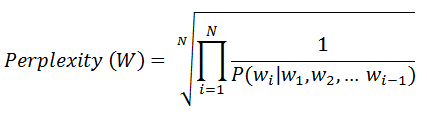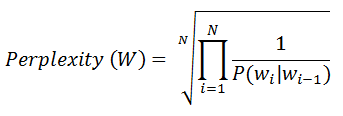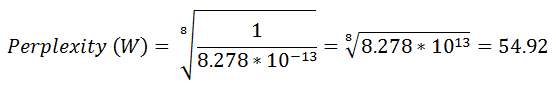Please visit, subscribe and share 10 Minutes Lectures in Computer Science

## Perplexity

In the context of Natural Language Processing (NLP), perplexity is a way to measure the quality of a language model independent of any application.

• Perplexity measures how well a probability model predicts the test data

• The model that assigns a higher probability to the test data is the better model. [A good model will assign a high probability to a real sentence]
• For example, let us assume that we estimate the probability of a test data using a bi-gram model and a tri-gram model. The better model among these is the one that has a tighter fit to the test data, or predicts the details of the test data better.

• Lower the perplexity, higher the probability

• Perplexity is an intrinsic evaluation metric (a metric that evaluates the given model independent of any application such as tagging, speech recognition etc.).

Formally, the perplexity is the function of the probability that the probabilistic language model assigns to the test data. For a test set W = w1, w2, …, wN, the perplexity is the probability of the test set, normalized by the number of words:Using the chain rule of probability, the equation can be expanded as follows;This equation can be modified to accommodate the language model that we use. For example, if we use a bigram language model, then the equation can be modified as follows;What is the value of N in this equation for a test set?

The test data can be a single sentence or a string consists of multiple sentences. Since this is the case, we need to include sentence boundary markers <s> and </s> in the probability estimation. Also, we need to include the end of sentence marker </s>, if any, in counting the total word tokens N. [Beginning of the sentence marker not include in the count as a token.]

Perplexity estimation – An example:
Let us suppose that as per a bigram model, the probability of a test sentence is as follows;
P(<s> Machine learning techniques learn the valuable patterns </s>) = 8.278*10-13.
Then the perplexity value for this model can be calculated as follows using the above equation;Here, N = 8. This includes 7 word tokens (Machine, learning, techniques, learn, the, valuable, patterns) with one end of sentence marker (</s>).

[Source: Speech and Language Processing by Daniel Jurafsky and James H. Martin]

*************

Go to Natural Language Processing (NLP) home

Go to NLP Glossary